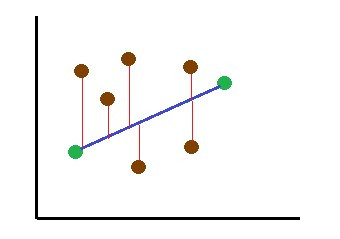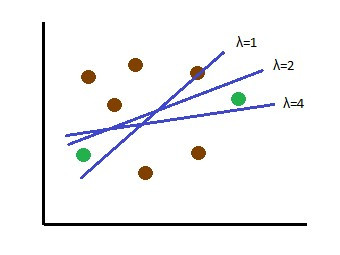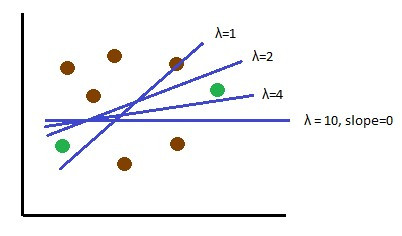Search

# Regularization in Linear ModelsIn machine learning, Regularization is a modification to be applied on a model so that we get more generalized model that fits well with new data.

Linear regression is an approach to find the relationship between variables using a straight line. It tries to find a line that best fits the data.Linear regression is simple and fast approach to find the relationship between variables but it tries to incorporate the noise/outliers also in the data. This may lead to less generalized model with more error while predicting the new data. So, to generalize the model, we regularize it.

When we talk about error, we commonly hear the terms Bias and Variance. So, let us first see what is bias and variance and then about Regularization techniques.

### Bias and Variance

When we predict data through a model, we get predicted values which may be at some distance from the actual values (distance is the deviation of predicted value from actual value). That distance is the error.

If the error we get is through passing training data to the model, it is called Bias.

If the error we get is through passing testing data to the model, it is called Variance.

A model which has less bias and less variance is considered as a good model because it can predict both training and testing data with less error.

### Sum of Squared Residuals in Linear Regression

A Residual is the difference between the predicted and actual value.

In linear regression, we predict a line to show the relationship between variables such that the sum of the squares of the residuals of the data points is less.In the above graph, green points are the data points and the red lines represent the residual. A line with less sum of squared residuals for the given data is considered as best fit line.

When we have only 2 training data points, then best fit line would be the line that pass through both the points in which case the sum of squared residuals is 0.But when we test this line with new data, we may get large sum of squared residuals for the new data.This means that this predicted line is having high variance. In other words, this line is overfitting the model.

### Ridge Regression

The Ridge regression also known as L2 regularization, is a regularization technique. This model finds a line that doesn’t fit the training data well but reduce the error with testing data. It means we introduce a small bias to the line fitting the data. But in return, we get drop in the variance.This new line called the Ridge regression line, provides better predictions than the previous line (linear model line) though this line doesn’t best fit the training data.

A regularized model finds a line that reduces sum of squared residuals added with a penalty. When the penalty is Sum of squared slopes then it is called Ridge regression.

So, Ridge regression reduces

### Sum of squared residuals + λ*Sum of (slopes) ^2

Here Sum of squared slopes is penalty and λ tells how severe that penalty is.

The value of λ can be anything between 0 and ∞(infinity).

When λ is 0 then

Sum of squared residuals + 0*Sum of (slopes) ^2 = Sum of squared residuals

So, when the λ is 0, the model tries to reduce only sum of the squared errors which gives linear regression line.

As the value of λ increase, the slope of the predicted line decreases.As λ is increased, the slope of the line gets asymptotically close to 0. So, when λ is large the dependency of output variable on the input features decreases. To find λ with least variance, we use cross-validation technique.

### Lasso Regression

Lasso Regression also known as L1 Regularization, is similar to Ridge regression. It also fits a line with little bias but which has less variance than linear regression. This is done by adding penalty to shrink the slope of the line until we get the best fit.

So, When the penalty of the model that regularizes is the Sum of absolute slopes then it is called Lasso regression.

So, Lasso regression reduces

### Sum of squared residuals + λ*(Sum of |slopes|)

Here the sum of absolute slopes is the penalty and λ tells how severe that penalty is.

The value of λ can be anything between 0 and ∞(infinity).

When λ is 0 then

Sum of squared residuals + 0*(Sum of |Slopes|) = Sum of squared residuals

So, when the λ is 0, the model tries to reduce only least squared errors which gives linear regression line.

As the value of λ increases, the slope of the predicted line decreases.So, as λ is increased, the slope of the line becomes 0. This is the main difference between Lasso and Ridge regression. While the Ridge regression reduce the slope very close to 0, the Lasso regression fully reduce it to 0.

Since Lasso regression can shrink slopes to 0, it removes the dependency of the output variable on some of the features. So, when our data contains many unimportant features, Lasso can do feature selection and make the dependency on these features to 0.

In contrast, Ridge regression works better when the output variable depends on all the features (it means, when all the features are important).

### Elastic Net Regression

When there are very large number of features and we don’t know which features are useful and which are not, then we can not choose between Lasso and Ridge Regressions. In this case, we can use Elastic net regression, which combines both Lasso and Ridge.

Elastic Net regression model tries to find a line that reduces

### Sum of squared residuals + λ1*(Sum of |slopes|) + λ2*Sum of (slopes) ^2

The λ1 and λ2 values are different. λ1 is for Lasso and λ2 is for Ridge. To get the best values for λ1 and λ2, we use cross-validation technique on different combination of values.

When both λ1, λ2 is 0 then we get linear regression line (line that only fits least sum of squared residuals).

When only λ1 is 0 then we get Ridge regression. When only λ2 is 0 then we get Lasso Regression.

### Conclusion

Regularization of a model deals with the over-fitting problem. There are many techniques for regularization. Choosing the best regularization method depends on the usefulness of the features. If all the features are important then the Ridge regression is chosen. If only some features are important to the output then Lasso Regression is used. When we have very large number of features and we don’t know about their usefulness then we choose Elastic Net Regression.